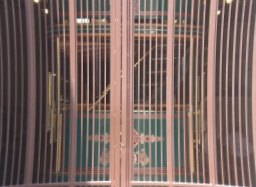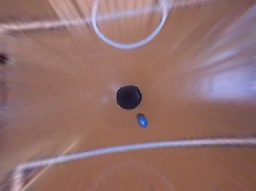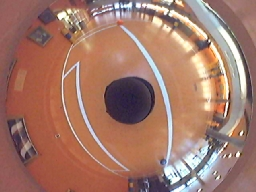The Radial Distortion module provides a way to correct for Barrel and Pincushion distortion often seen when capturing images with webcams or pinhole type cameras. This is also a necessary precursor to performing image measures that can be obscured by lens distortions. Note that once a corrected image is used the coefficients can be used on any other scene with that camera as the distortion coefficients are NOT content related but are related to the intrinsic camera properties.

## Interface## Instructions

1. Coefficients - specify the intrinsic camera coefficients. These can be obtained from the camera manufacturer or by experimentation. To manually correct use an image that has straight lines in it. For pincushion distortion the lines will bend outwards, for barrel distortion they will bend inwards. Adjust the coefficients until the lines appear straight.

2. You can use the slider to quick change coefficient R which will help you find the crude coefficients that can then be fine tuned using the above text boxes. Note that the higher order coefficients will normally be very very small.

3. Options Resampling - Select how you would like the transform to be performed. The Pixel Resample will use the closest pixel to the desired transform while the Bilinear method will use the pixel neighborhood to determine a new pixel value. The Pixel Resample is faster but less accurate. Most applications should use the Bilinear method unless performance is critical.

4. Crop Image - given a specific transform the image may contain areas that do not map correctly and will either appear as black borders or if the "Fill Edges" is on appear smeared. Selecting the "Crop Image" option will crop the image to a fully contained area. This has the unfortunate result of cropping actual image areas but is often not serious due to the minor amount of cropping.

5. Fill Edges - as apposed to cropping the image to a solid area you can chose to fill the unmapped edges to the closes border pixel. This will help to eliminate the dark borders but in extreme cases will appear to smear the image.

6.Display Grid Size - use to overlay a grid on the current image so that distortions become easier to see. Distortions will result in straight lines that appear bent. The grid draws many straight lines for you to compare with the lines in the image. Using this comparison you can better determine the optimal coefficients for the transform.

## Example

 Pincushion BarrelCorrectedYou can also use this module to unwrap 360 degree panoramic omnidirectional images.

 Original Undistorted## Polynomial

The following is a code snippet of how the distortion calculation works in order for you to better understand how the arguments are used. The following transforms a coordinate x,y into X,Y. Note that this module performs the inverse of this function in order to guarantee full pixel coverage.
```SCALE = 1000;
P0 = 1000.0; // order zero coefficient
PR = 1.0; // first order coefficient
PR2 = 0.0; // second order coefficient
PR3 = 0.0; // third order coefficient
PR4 = 0.0; // fourth order coefficient

r = sqrt(x^2+y^2);
z = P0 + PR*r + PR2*r*r + PR3*r*r*r + PR4*r*r*r*r;
s = -SCALE/z;

X = x*s;
Y = y*s;
```